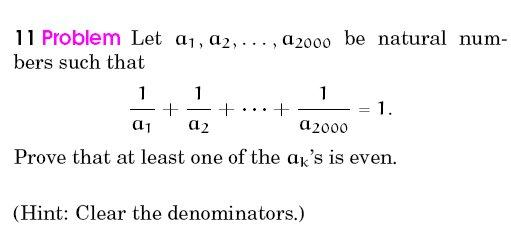# help me with this•Shubhodip ·

On the contrary assume none of them are even.

The given equality can be written as

a_2\cdot a_3 \cdots a_{2000} + a_1 \cdot a_3 \cdots a_{2000}+ \cdots + a_1\cdot a_2 \cdot a_{1999} = a_1 \cdot a_2 \cdot a_3 \cdots a_{2000}

In the RHS all the terms are odd, and are summed even i.e 2000 times. Hence RHS is even. But L.H.S is odd. Conclusion follows.

•rishabh ·

yeah. the best way to do these kind of Qs

•rahul ·

@pido- i couldn't get ur line "in RHS all the terms are odd and summed even times"... What does that mean?

•